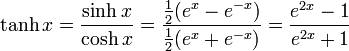getcarter

## Homework Statement

How to verify this trigonometric equation? tanh z/2?
verify that:

tanh (z/2) = [sinh x + i sin y] / [cosh x + cos y]

z is a complex number.

How can i verify this equation?

## Homework Equationshttp://mathworld.wolfram.com/images/equations/HyperbolicTangent/Inline3.gif
# sin(x + i y) = sin(x) cosh(y) + i cos(x) sinh(y).
# cos(x + i y) = cos(x) cosh(y) - i sin(x) sinh(y).
# tan(x + i y) = (tan(x) + i tanh(y)) / (1 - i tan(x) tanh(y)).
# cot(x + i y) = (cot(x) coth(y) - i) / (i cot(x) + coth(y)).

## The Attempt at a Solution

i have tried to solve from right hand side but it didn't work, then i have simplified the equation tanh(z/2) = e^z-1 / e^z+1

if you help me, i will be appreciate.
thanx a lot.